How To Solve a Complex DAX Problem - Excelerator BI

# How To Solve a Complex DAX Problem

I was helping someone on Power Pivot Forum last week with a tricky calculated column question.  As an aside, you may know that I always advise beginners to keep away from calculated columns.  You mostly don’t need calculated columns because in most circumstances a measure is a better option to use (read more about that here).  On this occasion however the required formula is likely to be complex, the run-time performance of such a formula as a measure could be slow, plus the number of possible unique values from the DAX Formula is small.  When these conditions are present, a calculated column can be a good solution.

## My Process is More Important Than My Solution

I want to say before starting that this blog article is actually about the process I went through to get a working solution.  I think the process is much more interesting and much more useful than the final formula.  In addition, when you look at the final formula you might think that you don’t even understand what the formula is doing let alone know how to write it.   But here is the killer point – when I look at the final formula I also think the same thing.  This is why the process of writing such a formula is so important.

### Here is the final formula

If you want to learn how to write such a formula then read on.

```=
CALCULATE(
MAX(Changes[new_value]),
FILTER(Changes, Changes[member_id] = EARLIER(Changes[member_id])),
FILTER(Changes, Changes[change_type] = "Type"),
FILTER(
Changes,
Changes[start_date]
= CALCULATE(
MAX(Changes[start_date]),
FILTER(
CALCULATETABLE(
Changes,
FILTER(Changes, Changes[member_id] = EARLIEST(Changes[member_id])),
FILTER(Changes, Changes[change_type] = "Type")
),
Changes[start_date] < EARLIEST(Changes[start_date])
)
)
)
)```

## The Problem Explained

There is a table of data (see sample below).  The table contains information about changes to membership status for members of a club.  At any point in time, a member has

• A status (active or inactive)
• A type (full, partial, limited)

Both “status” and “type” are indicated by a code.  The codes for status and type can and do change over time.  When these change, a change record is created that shows the old_value and the new_value.  One row in the table is equal to a single change.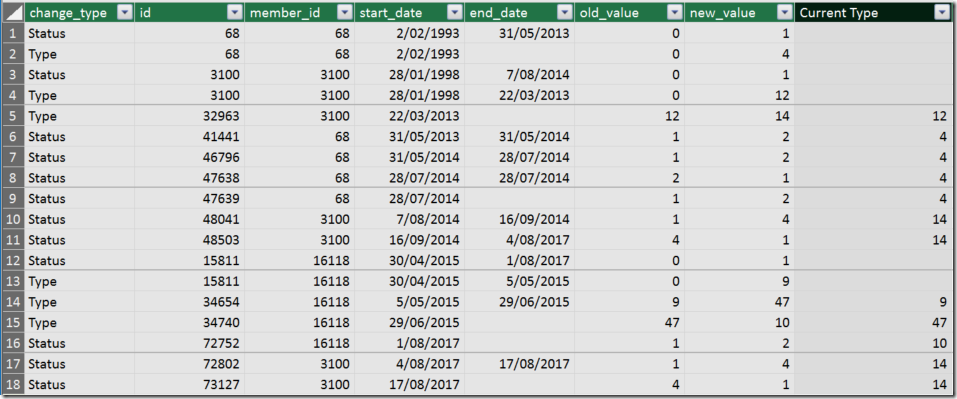### Key points are:

• The change record (row) can be for a change to the status or a change to the type as indicated in the Change_Type column.
• There is a Member_ID column that shows which member the change row belongs to.
• The date of the change is the start_date

### Objective

The objective is to know what the “current status” of the type code is for each member at the time the new change record was added.  Stated differently, at the time this change record was created, was this customer a full, partial or limited member?
The problem therefore is that I need to find the latest “type code” set prior to the date of the current transaction.  An example will make it clearer.

### Example

Here is a filtered copy of the table for member_id 3100.  The transactions are sorted in start_date order (which is the date of the transaction).  This table contains my final working calculated column formula “Current Type”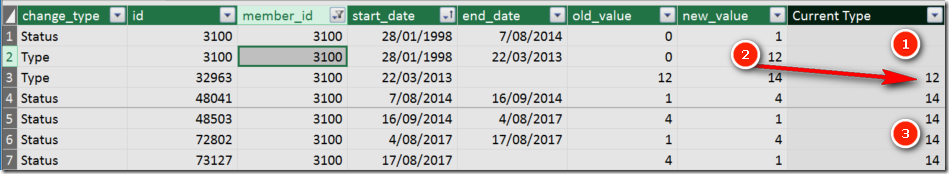• The first 2 transactions (rows) occurred on 28/1/1998 (start_date).  At this time there was no pre-existing entry for “Type” and as such the calculated column “Current Type” returns blank (see 1 above).
• The transaction in row 3 occurred on 22/3/2013 (start_date).  At this time the “Type” for this customer was “12” because this was the last code set as new_value prior to 22/3/2013.  In this case the “Type” was set on 28/1/1998 as shown in 2 above.  As a result, my calculated column returns 12 for record 3 (see 2 above).
• Record 3 actually changed the “Type” value from 12 to 14, so the next records (4 through 7) should return the value 14.  And they do as you can see in 3 above.

## A Different Design?

Before I share with you how I created this calculated column, it is worth pointing out that this probably would be easier to solve if there were 2 separate tables, one containing the “Type” transactions and another table containing the “Status” transactions.  Putting that aside, this is a great challenge as is, and working on challenging DAX problems will help you become better and stronger – a true DAX Ninja.

## How I Solved The Problem – Step by Step

### The Principle

I had a clear view in my mind what I needed to do before I started.  When solving any DAX problem, you need to think “filter first, evaluate second”.  In other words, filter the table I am given so it only contains what I need, then grab the values I am after.

With this in mind, for each record, I needed to:

1. Check which member the single record belonged to
2. Check the date of the change of this record
3. Filter the entire table for:
1. this one member
2. also for all records that were change_type  = “type”
3. also for all records prior to the current record date
4. Then once I had the above filtered table, I had to find the last record by start_date and then grab the number from the new_value column

### Step 1.  Get Started With Anything

Frankly I just wanted to write something that would get me started.  So I started with something simple.  I asked myself, “how many rows does each customer have in this table?”

I wrote this calculated column.

```=
CALCULATE(
COUNTROWS(Changes),
FILTER(Changes, Changes[member_id] = EARLIER(Changes[member_id]))
)```

Line 4 is the key.  Line 4 says…

“go and put a filter on the Changes table where the member_id is equal to the member_id of this row”.  The EARLIER function is needed because a calculated column has a row context and so does the FILTER function.

#### The VAR Syntax

If you have Excel 2016 or Power BI Desktop then you can use the VAR syntax.  The VAR syntax is actually easier to write and easier to read I think.  Here is the same formula with the VAR syntax.```=
VAR ThisMember = Changes[member_id]
RETURN
CALCULATE(
COUNTROWS(Changes),
FILTER(Changes, Changes[member_id] = ThisMember)
)```

I hope you agree that this VAR version is easier to read and understand.  First you set a value to the variable in line 2.  Then you can use this variable inside your formulas without having to worry about inner and outer row context.

OK, I am up and running and have solved the first problem. I have written a formula that correctly detects the current member_id and filters the table for that member (as you can see below in the WIP column).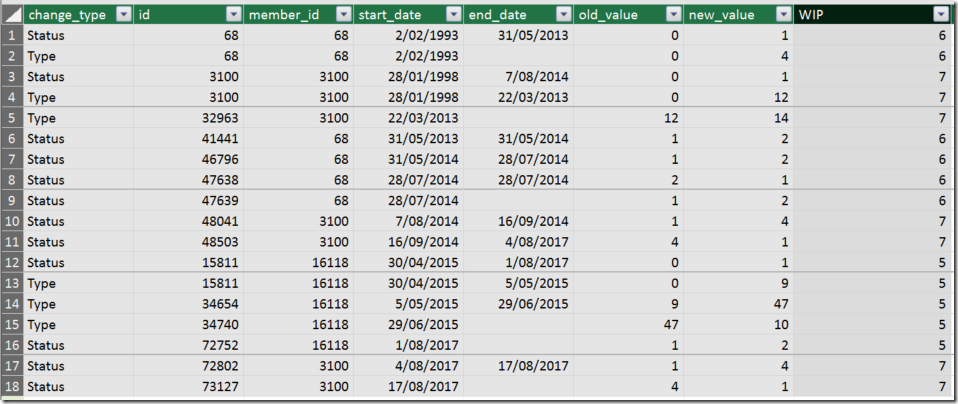Of course I needed to use COUNTROWS as it is not possible to put a table into a column.  Instead I used COUNTROWS as a trick so I could “see” how big the table is for each member.  I regularly use COUNTROWS when I am building complex formulas as it is one good way to “see” the virtual table I am building.  Another important point here is the test data.  When I first wrote this test formula I actually had 7 rows in the sample table for each customer.  This made it very difficult to check if the formula was working.  So before doing anything else I went back and changed my test data so that each member had a different number of rows.  Then I could “see” that the formula was working.  Good test data is essential to effectively write formulas.

### Step 2.  How Many Rows of “Type” for this Member?

Working through my high level mental plan, I decided to try to filter the table to count how many rows there were for each customer where the record was change_type = “type”.  Here is my new formula.

```=
CALCULATE(
COUNTROWS(Changes),
FILTER(Changes, Changes[member_id] = EARLIER(Changes[member_id])),
FILTER(Changes, Changes[change_type] = "Type")
)
```

As you can see I have switched back to the original syntax (without VAR).  I simply added a new filter on change_type = “type”.  It was easy to check if this formula was working by looking at the results.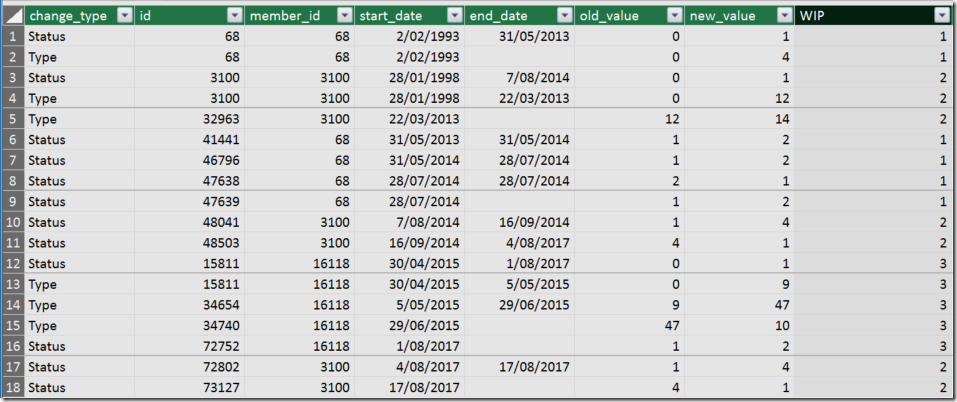### Step 3.  Extract Something More Useful

So far I have counted how many rows there are in these test tables.  So now that I had the correct table to use as my filter, I decided to try to extract the last start_date from this table.  This was as easy as changing line 3 as you can see below.

``` =
CALCULATE(
MAX(Changes[start_date]),
FILTER(Changes, Changes[member_id] = EARLIER(Changes[member_id])),
FILTER(Changes, Changes[change_type] = "Type")
)```

And the new formula now correctly extracts the last start_date of this table.  Remember the table contains all the change_type = “type” for this member_id, so this start_date is the date of the last “type” transaction found.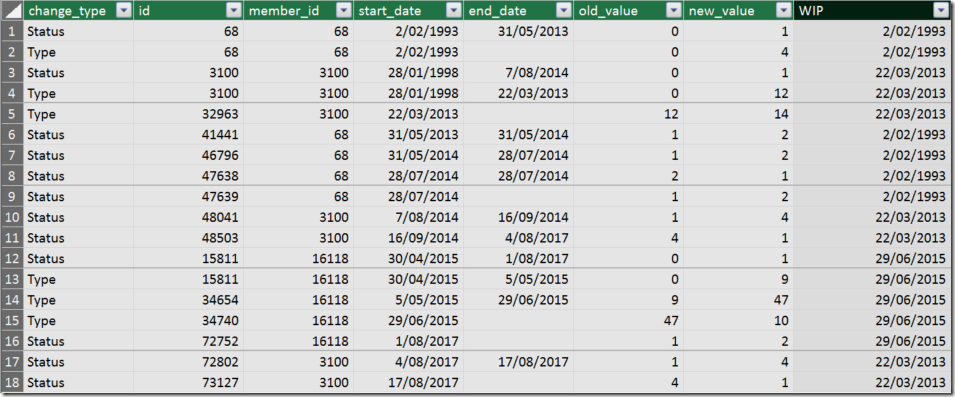### Step 4. Turn The 2 Filters into a Single Table

The above formula has 2 filter functions.  I really need it to be a single table so I can use it.  There are a few ways to do this, but I decided to change it into a table as follows.  Note I am still using COUNTROWS so I can validate it is still working.

```=
COUNTROWS(
CALCULATETABLE(
Changes,
FILTER(Changes, Changes[member_id] = EARLIER(Changes[member_id])),
FILTER(Changes,Changes[change_type] = "Type")
)
)```

Rows 3 to 7 now are a “table” that I can use as I move on.  Instead I could have used a single FILTER function with multiple filters using &&.

### Step 5. Grab the Last Date Before This Transaction Date

This table contains all type transactions.  But I don’t want all type transactions, I am looking for the last transaction that occurred before this current transaction.  In other words I need to filter this table to remove any type transactions that occurred on or after the date of this transaction.  I decided to switch back to the VAR syntax here to make it easier to write this formula.

```=
VAR thisStartDate = Changes[start_date]
RETURN
CALCULATE(
MAX(Changes[start_date]),
FILTER(
CALCULATETABLE(
Changes,
FILTER(Changes, Changes[member_id] = EARLIER(Changes[member_id])),
FILTER(Changes, Changes[change_type] = "Type")
),
Changes[start_date] < thisStartDate
)
)```

Note above rows 7 – 11 are the table I produced in step 4.  I wrapped this inside another FILTER to remove all records on or after the date of the current record, only keeping records that occurred before the date of the current change record.  Rows 6 through 13 therefore returns a table that contains all ‘type’ records that occurred before this record.  I then use the CALCULATE statement to return the date of the last type transaction.  As you will see in the resulting table below, the WIP column now returns blanks for any record that doesn’t have a ‘type’ record for an earlier period in time.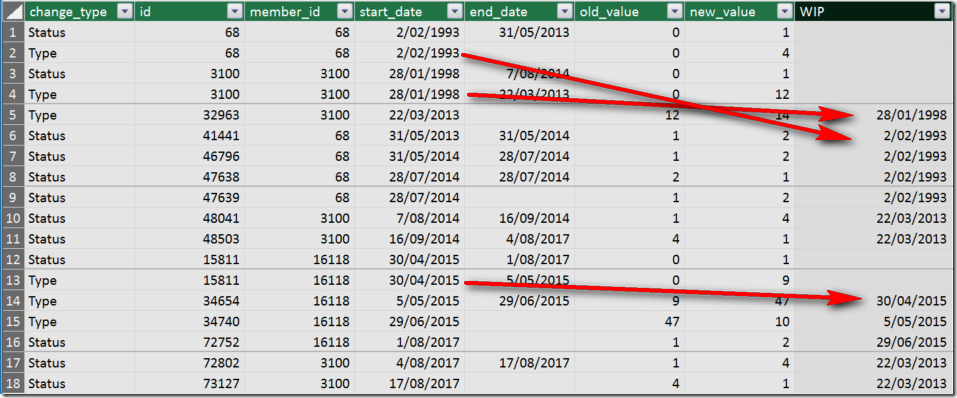### Step 6.  Extract the Value from the Previous “Type” Record

Now I know the date of the correct ‘type’ record, I can proceed to extract the value of that record from the new_value column.  Here is my formula.

```=
VAR thisStartDate = Changes[start_date]
VAR dateToUse =
CALCULATE(
MAX(Changes[start_date]),
FILTER(
CALCULATETABLE(
Changes,
FILTER(Changes, Changes[member_id] = EARLIER(Changes[member_id])),
FILTER(Changes, Changes[change_type] = "Type")
),
Changes[start_date] < thisStartDate)
)
RETURN
CALCULATE(
MAX(Changes[new_value]),
FILTER(Changes, Changes[member_id] = EARLIER(Changes[member_id])),
FILTER(Changes, Changes[change_type] = "Type"),
Changes[start_date] = dateToUse
)```

Note how I have taken the result of the formula in step 5 and turned that into another VAR (rows 3- 14).  Then I have written a new formula (rows 16 – 21 above) to extract the value I need after filtering for the member_id (row 18), the change_type (row 19) and the correct date of the transaction (row 20).## One Last Complication

I did have one last problem that I came across.  The OP at http://powerpivotforum.com.au was using Excel 2010, so I couldn’t provide the above formula (Excel 2010 doesn’t support the VAR syntax).  So I had to remove the VAR syntax.  First I removed the first VAR (line 2 from step 6 above) as follows.  Note how I had to use EARLIER in line 11 to manage the row context.

```=
VAR dateToUse =
CALCULATE(
MAX(Changes[start_date]),
FILTER(
CALCULATETABLE(
Changes,
FILTER(Changes, Changes[member_id] = EARLIER( Changes[member_id])),
FILTER(Changes, Changes[change_type] = "Type")
),
Changes[start_date] < EARLIER(Changes[start_date])
)
)
RETURN
CALCULATE(
MAX(Changes[new_value]),
FILTER(Changes, Changes[member_id] = EARLIER(Changes[member_id])),
FILTER(Changes, Changes[change_type] = "Type"),
FILTER(Changes, Changes[start_date] = dateToUse)
)```

Finally I removed the second VAR from step 6 as follows.  I grabbed lines 3 -13 from the formula above, and replaced dateToUse above in line 19 with this formula.

```=
CALCULATE(
MAX(Changes[new_value]),
FILTER(Changes, Changes[member_id] = EARLIER(Changes[member_id])),
FILTER(Changes, Changes[change_type] = "Type"),
FILTER(
Changes,
Changes[start_date]
= CALCULATE(
MAX(Changes[start_date]),
FILTER(
CALCULATETABLE(
Changes,
FILTER(Changes, Changes[member_id] = EARLIEST(Changes[member_id])),
FILTER(Changes, Changes[change_type] = "Type")
),
Changes[start_date] < EARLIEST(Changes[start_date])
)
)
)
)
```

After making this change, note that this time in lines 14 and 17 I had to tweak the formula to correctly manage the nested row context, but this time I had to refer to EARLIEST instead of EARLIER.  This is because there are 3 nested sets of row context.  The first is the calculated column, the second is the FILTER on row 6 and the third is the FILTER on row 11.

## A Final Word

The key point to this post is that the final formula above is hard to read and understand.  You may think that this formula is written starting at the top and working to the bottom, but this is far from reality.  Complex DAX formulas rarely (if ever) are written top down.  The trick to writing complex DAX formulas is to break the problem into pieces and solve each piece of the puzzle one step at a time.

Here is the sample_workbook and here are all the formulas I wrote.

## Do You have a Better Solution?

I know there are some talented DAX Ninjas out there that read my blog (Jess, Owen to name 2).  I always love learning so if you have a better formula/solution or interesting alternative approach than this I would love to see it.  Please post in the comments.

### 27 thoughts on “How To Solve a Complex DAX Problem”

1.see my code- This runs fine./
calculate(max(Table1[new value]),TOPN(1,FILTER(filter(filter(Table1,Table1[change type]=”type”),Table1[id]=earlier(Table1[id])),Table1[date]<earlier(Table1[date])),Table1[date],DESC))

NB- Table1 is the name of table. Id is Member_id.Date is Start_Date. Fo convinience I changed.
Less code, enjoy life. If you have ny thought, let me know.

2.Darryl Wolfaardt

I cannot thank you enough. Showing HOW to think through a problem is so much more valuable than just solving it.

3.…sorry – think I solved it – I had pasted it into a new Measure – still trying to work out how EARLIER works but I’ll get it soon!

4.Thanks Matt – thought Id give this a go. Pasting in one of your DAX in Power BI after loading in the table just errors every time I use EARLIER
MYMEASURE = CALCULATE(COUNTROWS(Changes),filter(Changes,Changes[member_id]=EARLIER(Changes[member_id])))
“EARLIER/EARLIEST refers to an earlier row context which doesn’t exist.”. Am I missing some point? maybe my Power BI is too late for your article. Id really like to go through it as its got some awkward syntax Id like to be more comfortable with. Especially EARLIER 🙂
Thanks

5.Aleksey Semiletov

Thanks for the post, Matt.
Great article! It helped me to solve the similar problem.
But if in the table filtered by the change_type and member_id you’ll see the rows with the same start_date? I think it’ll broke the formula. It’s rare example of data, but it can happen.
Sorry for my English, it’s not good.

1.If it is possible to have 2 changes on the same date for the same member ID, then any human looking at the 2 records would not know which is the latest. As a result, no formula could do it either (with these data). So presumably if this were possible, the date column would have a time stamp, or alternatively there would need to be a transaction iD.

6.in this moment i have a bit problem in dax, for me is big problem, but i can´t find a solution

7.Hi Matt, my name is Eduardo, i am from Ecuador, I speak english so so, i buyed your book, its great

8.Hi Matt –

Great article, love seeing how you step through the process.

Overall, I think your measure performs pretty well. For the most part your FILTER() calls are optimized in Vertipaq, so I don’t see much need for tuning.

My only suggestion is to prebuild tables as variables. I just learned you could do this, and I think it really helps to read a DAX formula as “top down”. This way each piece of the puzzle is defined first, and the final return statement is simple to read and reference.
I built this in http://www.daxformatter.com, and I’m trying to paste the “Copy HTML” results, we’ll see if it works:

New Column =/*This formula requires Excel 2016 or Power BI, since it uses VAR syntax
Written by Chris H on 9/6/2017*///Same base variables as beforeVAR ThisMember = Changes[member_id]VAR thisStartDate = Changes[start_date] /* This base table is referenced twice, so it’s faster to create it once as a variable*/VAR TableMemberType =    FILTER (        Changes,        Changes[member_id] = ThisMember            && Changes[change_type] = “Type”    ) //This is the same variable as before, but it’s referencing the new table variableVAR dateToUse =    CALCULATE (        MAX ( Changes[start_date] ),        FILTER ( TableMemberType, Changes[start_date] < thisStartDate )    ) //Another new table based on the the first table variable, just adding a new filter to the stackVAR TableMemberTypeDate =    FILTER ( TableMemberType, Changes[start_date] = dateToUse )RETURN    //The returned calculation looks much cleaner since the table has been previously defined    CALCULATE (        MAX ( Changes[new_value] ),        TableMemberTypeDate    )

If it didn’t, the plain formatted text is as follows:

New Column =
/*This formula requires Excel 2016 or Power BI, since it uses VAR syntax
Written by Chris H on 9/6/2017*/
//Same base variables as before
VAR ThisMember = Changes[member_id]
VAR thisStartDate = Changes[start_date] /* This base table is referenced twice, so it’s faster to create it once as a variable*/
VAR TableMemberType =
FILTER (
Changes,
Changes[member_id] = ThisMember
&& Changes[change_type] = “Type”
) //This is the same variable as before, but it’s referencing the new table variable
VAR dateToUse =
CALCULATE (
MAX ( Changes[start_date] ),
FILTER ( TableMemberType, Changes[start_date] < thisStartDate )
) //Another new table based on the the first table variable, just adding a new filter to the stack
VAR TableMemberTypeDate =
FILTER ( TableMemberType, Changes[start_date] = dateToUse )
RETURN
//The returned calculation looks much cleaner since the table has been previously defined
CALCULATE (
MAX ( Changes[new_value] ),
TableMemberTypeDate
)

1.Tables as a var is an excellent idea but not possible given the user had 2010. But I do plan to post on var in the future.

9.Thank you Matt for showing the process, it’s why I read all your articles. I really like the variable approach, it’s so much clearer not just when learning, but when going back and trying to troubleshoot later when something inevitably changes.

10.This is great Matt.
Only comment is that you can use a variable also instead of EARLIER() , if you are using supporting versions, to make the code a bit more readable, since you either way use variables.

VAR ThisMember = Changes[member_id]

1.You are right Konstantinos, and this is already covered in the article.

11.Came for the process and wasn’t disappointed, thanks for the detailed write-up Matt; breaking down your approach to attacking more complex DAX formulas is very valuable to us DAX newbies.

12.Thanks Matt this is fantastic both regarding the thought process and the actual DAX technicalities.

I work in Education and Student and Teachers change groups regularly and my current method was to just record the snapshot data @ the time of Collection which obviously bloats the table. This method should enable me just to use the change records.

13.Matt thanks for sharing. Agreed that the process is more important than the final formula. Once you have a working formula then you can fine tune it if you are concerned about performance.

14.Very similar to the monster formulae phenomenon in Excel. At least you have the ability here to use DAX formatter and some commentary so the logic is documented. That means anyone else peeling back the layers at least has a chance
It all depends on who is going to inherit It i suppose
I’m not averse to using a couple of calculated columns to simplify DAX if they purely serve to be ‘helpers’ and are not performance hungry

15.Thanks for the post Matt – great to read through your problem-solving process!

In situations like this, I would use something like below.
Here I have used CALCULATE to create a filter context corresponding to the current row, then ALLEXCEPT to discard all the filters except the one I want to keep (member_id in this case), plus added the other start_date and change_type filters.
In this case we want the last date within this context so I used a second CALCULATE(…,LASTDATE(…)) within the outer CALCULATE.

=
CALCULATE (
CALCULATE ( MAX ( Changes[new_value] ), LASTDATE ( Changes[start_date] ) ),
ALLEXCEPT ( Changes, Changes[member_id] ),
Changes[start_date] < EARLIER ( Changes[start_date] ),
Changes[change_type] = "Type"
)

1.Nice Owen – I knew I could rely on you!

1.Hi @Matt, @Owen,

I like your blog posts very much Matt and Owen's idea was magnificent I'm not sure I would have thought of that approach.

2.Excellent, Owen! 🙂 I managed almost the same solution, except I used LASTNONBLANK (and it looks excessive) instead of MAX:
=
CALCULATE (
CALCULATE (
LASTNONBLANK ( Changes[new_value], 1 ),
LASTDATE ( Changes[start_date] )
),
ALLEXCEPT ( ‘Changes’, Changes[member_id] ),
Changes[start_date] < EARLIER ( Changes[start_date] ),
Changes[change_type] = "Type"
)

16.Hi Matt,

Would the following achieve the same result?:
=CALCULATE(MAX(Changes[new_value]),
FILTER(Changes,Changes[member_id] = EARLIER(Changes[member_id])),
FILTER(Changes,Changes[change_type] = “Type”),
FILTER(Changes,Changes[start_date] = CALCULATE(MAX(Changes[start_date]),
FILTER(Changes,Changes[member_id] = EARLIEST(Changes[member_id])),
FILTER(Changes,Changes[change_type] = “Type”),
FILTER(Changes,Changes[start_date] < EARLIEST(Changes[start_date]))
)
)
)

It does in the sample workbook but obviously that is not the entire data set.

1.They look conceptually the same to me, it is just the timing of when the filters are applied.

17.Matt. you approach to solve a complex problem is incredible.
Thanks for sharing the process and the formulas.

1.Max kaka nam he uska… Kaka lagta he wo apna.

Scroll to Top